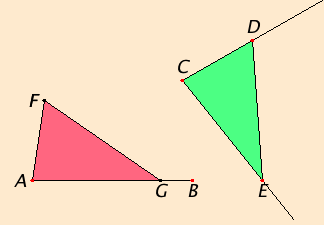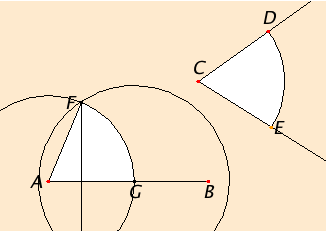# Proposition 23

To construct a rectilinear angle equal to a given rectilinear angle on a given straight line and at a point on it.

Let the angle DCE be the given rectilinear angle, AB the given straight line, and A the point on it.It is required to construct a rectilinear angle equal to the given rectilinear angle DCE on the given straight line AB and at the point A on it.

Take the points D and E at random on the straight lines CD and CE respectively, and join DE. Out of three straight lines which equal the three straight lines CD, DE, and CE construct the triangle AFG in such a way that CD equals AF, CE equals AG, and DE equals FG.

I.8

Since the two sides DC and CE equal the two sides FA and AG respectively, and the base DE equals the base FG, therefore the angle DCE equals the angle FAG.

Therefore on the given straight line AB, and at the point A on it, the rectilinear angle FAG has been constructed equal to the given rectilinear angle DCE.

Q.E.F.

## Guide

As Proclus and Heath point out, a very minor variant of the construction in I.22 is needed to make the triangle AFG. The problem is that in I.22 the triangle is placed not at the end of line, but somewhere beyond that, and in I.23, the triangle needs to be placed right at the end A of the line.

#### Construction steps

 This construction that moves an angle requires a number of steps involving a straightedge and compass. Unless there is some special reason for selecting particular points D and E on the sides of the angle C, they might as well be taken equidistant from C as Apollonius suggested. Then only two distances need to be transferred instead of three. In order to make CE equal to CD, one circle is required. Next, in order to transfer the distance CD to A, four circles (not shown) are required as per Propositions I.2 and I.1, and four more circles (also not shown) to transfer ED to G. Finally, two more circles are required, one with center A and radius CD (which has been transferred), the other with center G and radius ED. These last two circles meet at the point F, and the line AF is the other side of the required angle.In all there are ten circles and one line that must be drawn. The lines in the intermediate stages may be suppressed as usual since they’re only needed to verify the construction is correct.

#### Use of Proposition 23

The construction in this proposition is used in the next one and a couple others in Book I. It is also used frequently in the later books. It is also used frequently in BookS III and VI and occasionally in Books IV and XI.

Although it may appear that the triangles are to be in the same plane, that is not necessary. Indeed, the construction in this proposition is used to construct an angle in a different plane in proposition XI.31.